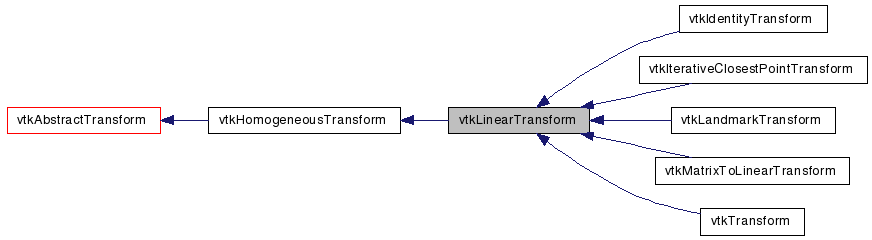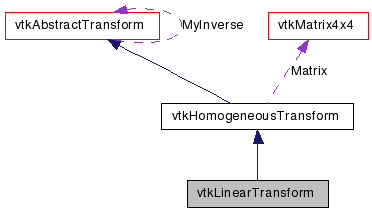# vtkLinearTransform Class Reference

`#include <vtkLinearTransform.h>`

Inheritance diagram for vtkLinearTransform:[legend]
Collaboration diagram for vtkLinearTransform:[legend]

## Detailed Description

abstract superclass for linear transformations

vtkLinearTransform provides a generic interface for linear (affine or 12 degree-of-freedom) geometric transformations.

vtkTransform vtkIdentityTransform

Definition at line 29 of file vtkLinearTransform.h.

## Public Types

typedef vtkHomogeneousTransform Superclass

## Public Member Functions

virtual const char * GetClassName ()
virtual int IsA (const char *type)
void PrintSelf (ostream &os, vtkIndent indent)
void TransformPoints (vtkPoints *inPts, vtkPoints *outPts)
virtual void TransformNormals (vtkDataArray *inNms, vtkDataArray *outNms)
virtual void TransformVectors (vtkDataArray *inVrs, vtkDataArray *outVrs)
void TransformNormal (const float in, float out)
void TransformNormal (const double in, double out)
double * TransformNormal (double x, double y, double z)
double * TransformNormal (const double normal)
float * TransformFloatNormal (float x, float y, float z)
float * TransformFloatNormal (const float normal)
double * TransformDoubleNormal (double x, double y, double z)
double * TransformDoubleNormal (const double normal)
double * TransformVector (double x, double y, double z)
double * TransformVector (const double normal)
void TransformVector (const float in, float out)
void TransformVector (const double in, double out)
float * TransformFloatVector (float x, float y, float z)
float * TransformFloatVector (const float vec)
double * TransformDoubleVector (double x, double y, double z)
double * TransformDoubleVector (const double vec)
void TransformPointsNormalsVectors (vtkPoints *inPts, vtkPoints *outPts, vtkDataArray *inNms, vtkDataArray *outNms, vtkDataArray *inVrs, vtkDataArray *outVrs)
vtkLinearTransformGetLinearInverse ()
void InternalTransformPoint (const float in, float out)
void InternalTransformPoint (const double in, double out)
virtual void InternalTransformNormal (const float in, float out)
virtual void InternalTransformNormal (const double in, double out)
virtual void InternalTransformVector (const float in, float out)
virtual void InternalTransformVector (const double in, double out)
void InternalTransformDerivative (const float in, float out, float derivative)
void InternalTransformDerivative (const double in, double out, double derivative)

## Static Public Member Functions

static int IsTypeOf (const char *type)
static vtkLinearTransformSafeDownCast (vtkObject *o)

## Protected Member Functions

vtkLinearTransform ()
~vtkLinearTransform ()

## Member Typedef Documentation

Reimplemented from vtkHomogeneousTransform.

Definition at line 33 of file vtkLinearTransform.h.

## Constructor & Destructor Documentation

 vtkLinearTransform::vtkLinearTransform ( ) ` [inline, protected]`

Definition at line 197 of file vtkLinearTransform.h.

 vtkLinearTransform::~vtkLinearTransform ( ) ` [inline, protected]`

Definition at line 198 of file vtkLinearTransform.h.

## Member Function Documentation

 virtual const char* vtkLinearTransform::GetClassName ( ) ` [virtual]`

Reimplemented from vtkHomogeneousTransform.

 static int vtkLinearTransform::IsTypeOf ( const char * name ) ` [static]`

Return 1 if this class type is the same type of (or a subclass of) the named class. Returns 0 otherwise. This method works in combination with vtkTypeMacro found in vtkSetGet.h.

Reimplemented from vtkHomogeneousTransform.

 virtual int vtkLinearTransform::IsA ( const char * name ) ` [virtual]`

Return 1 if this class is the same type of (or a subclass of) the named class. Returns 0 otherwise. This method works in combination with vtkTypeMacro found in vtkSetGet.h.

Reimplemented from vtkHomogeneousTransform.

 static vtkLinearTransform* vtkLinearTransform::SafeDownCast ( vtkObject * o ) ` [static]`

Reimplemented from vtkHomogeneousTransform.

 void vtkLinearTransform::PrintSelf ( ostream & os, vtkIndent indent ) ` [virtual]`

Methods invoked by print to print information about the object including superclasses. Typically not called by the user (use Print() instead) but used in the hierarchical print process to combine the output of several classes.

Reimplemented from vtkHomogeneousTransform.

 void vtkLinearTransform::TransformNormal ( const float in, float out ) ` [inline]`

Apply the transformation to a normal. You can use the same array to store both the input and output.

Definition at line 39 of file vtkLinearTransform.h.

 void vtkLinearTransform::TransformNormal ( const double in, double out ) ` [inline]`

Apply the transformation to a double-precision normal. You can use the same array to store both the input and output.

Definition at line 46 of file vtkLinearTransform.h.

 double* vtkLinearTransform::TransformNormal ( double x, double y, double z ) ` [inline]`

Synonymous with TransformDoubleNormal(x,y,z). Use this if you are programming in python, tcl or Java.

Definition at line 53 of file vtkLinearTransform.h.

 double* vtkLinearTransform::TransformNormal ( const double normal ) ` [inline]`

Synonymous with TransformDoubleNormal(x,y,z). Use this if you are programming in python, tcl or Java.

Definition at line 55 of file vtkLinearTransform.h.

 float* vtkLinearTransform::TransformFloatNormal ( float x, float y, float z ) ` [inline]`

Apply the transformation to an (x,y,z) normal. Use this if you are programming in python, tcl or Java.

Definition at line 62 of file vtkLinearTransform.h.

 float* vtkLinearTransform::TransformFloatNormal ( const float normal ) ` [inline]`

Apply the transformation to an (x,y,z) normal. Use this if you are programming in python, tcl or Java.

Definition at line 68 of file vtkLinearTransform.h.

 double* vtkLinearTransform::TransformDoubleNormal ( double x, double y, double z ) ` [inline]`

Apply the transformation to a double-precision (x,y,z) normal. Use this if you are programming in python, tcl or Java.

Definition at line 75 of file vtkLinearTransform.h.

 double* vtkLinearTransform::TransformDoubleNormal ( const double normal ) ` [inline]`

Apply the transformation to a double-precision (x,y,z) normal. Use this if you are programming in python, tcl or Java.

Definition at line 81 of file vtkLinearTransform.h.

 double* vtkLinearTransform::TransformVector ( double x, double y, double z ) ` [inline]`

Synonymous with TransformDoubleVector(x,y,z). Use this if you are programming in python, tcl or Java.

Definition at line 88 of file vtkLinearTransform.h.

 double* vtkLinearTransform::TransformVector ( const double normal ) ` [inline]`

Synonymous with TransformDoubleVector(x,y,z). Use this if you are programming in python, tcl or Java.

Definition at line 90 of file vtkLinearTransform.h.

 void vtkLinearTransform::TransformVector ( const float in, float out ) ` [inline]`

Apply the transformation to a vector. You can use the same array to store both the input and output.

Definition at line 97 of file vtkLinearTransform.h.

 void vtkLinearTransform::TransformVector ( const double in, double out ) ` [inline]`

Apply the transformation to a double-precision vector. You can use the same array to store both the input and output.

Definition at line 104 of file vtkLinearTransform.h.

 float* vtkLinearTransform::TransformFloatVector ( float x, float y, float z ) ` [inline]`

Apply the transformation to an (x,y,z) vector. Use this if you are programming in python, tcl or Java.

Definition at line 111 of file vtkLinearTransform.h.

 float* vtkLinearTransform::TransformFloatVector ( const float vec ) ` [inline]`

Apply the transformation to an (x,y,z) vector. Use this if you are programming in python, tcl or Java.

Definition at line 117 of file vtkLinearTransform.h.

 double* vtkLinearTransform::TransformDoubleVector ( double x, double y, double z ) ` [inline]`

Apply the transformation to a double-precision (x,y,z) vector. Use this if you are programming in python, tcl or Java.

Definition at line 124 of file vtkLinearTransform.h.

 double* vtkLinearTransform::TransformDoubleVector ( const double vec ) ` [inline]`

Apply the transformation to a double-precision (x,y,z) vector. Use this if you are programming in python, tcl or Java.

Definition at line 130 of file vtkLinearTransform.h.

 void vtkLinearTransform::TransformPoints ( vtkPoints * inPts, vtkPoints * outPts ) ` [virtual]`

Apply the transformation to a series of points, and append the results to outPts.

Reimplemented from vtkHomogeneousTransform.

Reimplemented in vtkIdentityTransform.

 virtual void vtkLinearTransform::TransformNormals ( vtkDataArray * inNms, vtkDataArray * outNms ) ` [virtual]`

Apply the transformation to a series of normals, and append the results to outNms.

Reimplemented in vtkIdentityTransform.

 virtual void vtkLinearTransform::TransformVectors ( vtkDataArray * inVrs, vtkDataArray * outVrs ) ` [virtual]`

Apply the transformation to a series of vectors, and append the results to outVrs.

Reimplemented in vtkIdentityTransform.

 void vtkLinearTransform::TransformPointsNormalsVectors ( vtkPoints * inPts, vtkPoints * outPts, vtkDataArray * inNms, vtkDataArray * outNms, vtkDataArray * inVrs, vtkDataArray * outVrs ) ` [virtual]`

Apply the transformation to a combination of points, normals and vectors.

Reimplemented from vtkHomogeneousTransform.

Reimplemented in vtkIdentityTransform.

 vtkLinearTransform* vtkLinearTransform::GetLinearInverse ( ) ` [inline]`

Just like GetInverse, but it includes a typecast to vtkLinearTransform.

Definition at line 160 of file vtkLinearTransform.h.

 void vtkLinearTransform::InternalTransformPoint ( const float in, float out ) ` [virtual]`

This will calculate the transformation without calling Update. Meant for use only within other VTK classes.

Reimplemented from vtkHomogeneousTransform.

Reimplemented in vtkIdentityTransform.

 void vtkLinearTransform::InternalTransformPoint ( const double in, double out ) ` [virtual]`

This will calculate the transformation without calling Update. Meant for use only within other VTK classes.

Reimplemented from vtkHomogeneousTransform.

Reimplemented in vtkIdentityTransform.

 virtual void vtkLinearTransform::InternalTransformNormal ( const float in, float out ) ` [virtual]`

This will calculate the transformation without calling Update. Meant for use only within other VTK classes.

Reimplemented in vtkIdentityTransform.

 virtual void vtkLinearTransform::InternalTransformNormal ( const double in, double out ) ` [virtual]`

This will calculate the transformation without calling Update. Meant for use only within other VTK classes.

Reimplemented in vtkIdentityTransform.

 virtual void vtkLinearTransform::InternalTransformVector ( const float in, float out ) ` [virtual]`

This will calculate the transformation without calling Update. Meant for use only within other VTK classes.

Reimplemented in vtkIdentityTransform.

 virtual void vtkLinearTransform::InternalTransformVector ( const double in, double out ) ` [virtual]`

This will calculate the transformation without calling Update. Meant for use only within other VTK classes.

Reimplemented in vtkIdentityTransform.

 void vtkLinearTransform::InternalTransformDerivative ( const float in, float out, float derivative ) ` [virtual]`

This will calculate the transformation as well as its derivative without calling Update. Meant for use only within other VTK classes.

Reimplemented from vtkHomogeneousTransform.

Reimplemented in vtkIdentityTransform.

 void vtkLinearTransform::InternalTransformDerivative ( const double in, double out, double derivative ) ` [virtual]`

This will calculate the transformation as well as its derivative without calling Update. Meant for use only within other VTK classes.

Reimplemented from vtkHomogeneousTransform.

Reimplemented in vtkIdentityTransform.

The documentation for this class was generated from the following file:

Generated on Wed Aug 24 11:45:55 2011 for VTK by1.5.6